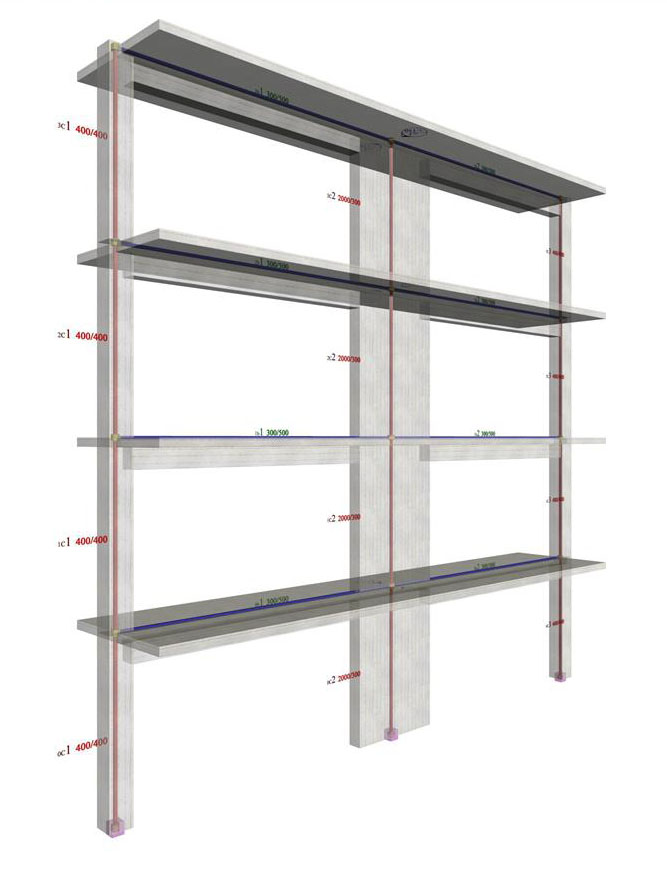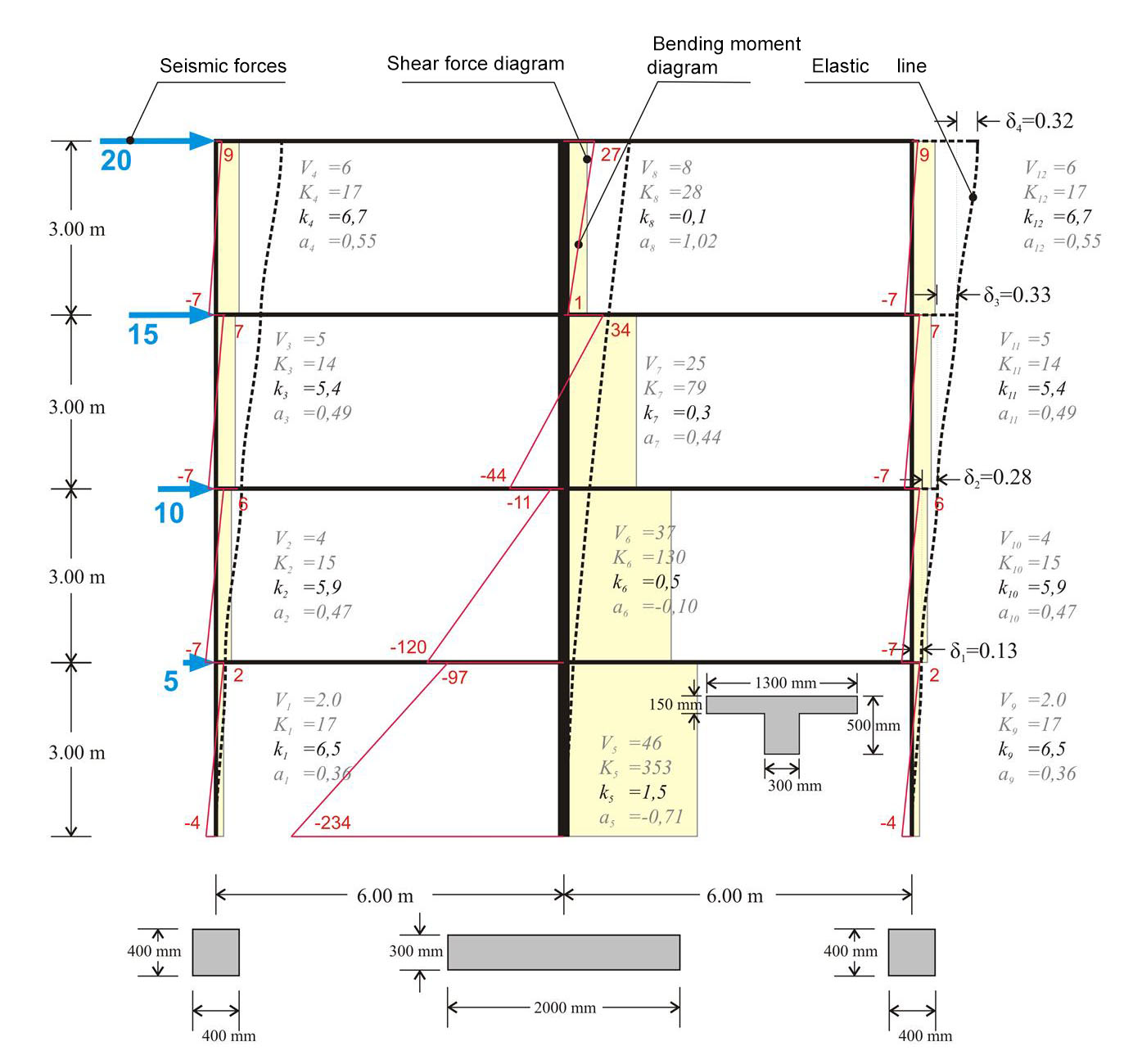« Multistorey plane frame systems Comparison of multistorey frame and dual systems » Multistorey plane dual systems In the following multistorey plane frame of project the interstorey fluctuation of the apparent stiffness factors kj and the moment distribution factor aj in both columns and wall is presented.Figure 5.3.2-1: Four-storey dual type structure comprising two columns and a wall Figure 5.3.2-1: Four-storey dual type structure comprising two columns and a wall If the frame also comprises walls, the differences in stiffnesses and in moment distributions become more intense as the number of stories increase.Figure 5.3.2-2: Multistorey dual type structure with triangular distribution of seismic forces( "hand-made" graphical representation) Figure 5.3.2-2: Multistorey dual type structure with triangular distribution of seismic forces( "hand-made" graphical representation) Example 5.3.2 (3rd storey) : Column stiffnesses: K3,1=4.6/0.329=14, K3,2=25.9/0.329=79 K3,3=4.6/0.329=14. Storey stiffness: Κ 3 = (20+15)/0.329=107. The apparent relative stiffness of the storey can also derive from the sum of its column stiffnesses, i.e. K3= K3,1+K3,2+ K3,3=107. If shear effect is taken into account (Shear effect=ON), the displacements are δ 1 =0.14, δ 2 =0.30, δ 3 =0.34, δ 4 =0.32 mm, i.e. the difference is small. If, however, rigid bodies effect is taken into account (Rigid body=ON), the displacements obtained are significantly smaller equal to δ 1 =0.12, δ 2 =0.22, δ 3 =0.24, δ 4 =0.21 mm, leading to much larger stiffness values. In case of a basement, zero seismic forces are developed at its roof level. However the fixed end condition applies at the bases only of the columns located at the basement perimeter walls. In the multistorey dual frame, the column factors are characterized by relatively small fluctuations with k varying from 5 to 7 and a from 0.35 to 0.55. On the contrary the corresponding fluctuation in the wall factors is significant with k varying from 0.10 to 1.50 and a from 1.0 to -1.0 [*]NoteIn case the frame has more storeys, the value of factor k decreases further. It should be noted that it could reach even negative values in the upper storeys. In such cases walls have an unfavourable effect on frames. Consequently, it is therefore pointed out, that although walls behave in a relieving way in the lower storeys, in the upper ones they might even behave in a negative way. To this end it is recommended to use dual systems in multistorey buildings. In dual systems, walls function effectively in the lower storeys, while frames in the upper ones. . « Multistorey plane frame systems Comparison of multistorey frame and dual systems »